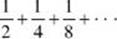﻿ ﻿SERIES - Sequences and Series - Numbers and Operations - REVIEW OF MAJOR TOPICS - SAT SUBJECT TEST MATH LEVEL 2

## PART 2## REVIEW OF MAJOR TOPICS## Numbers and Operations

###3.4 Sequences and Series### SERIES

In a geometric sequence, if |r| < 1, the sum of the series approaches a limit as n approaches infinity. In the formulathe termTherefore, as long as, orEXAMPLES

1. Evaluate (A)and

(B)Both problems ask the same question: Find the sum of an infinite geometric series.

(A) When the first few terms,, are listed, it can be seen thatand the common ratio. Therefore,(B) When the first few terms,, are listed, it can be seen that t1 = 1 and the common ratio. Therefore,2. Find the exact value of the repeating decimal 0.4545 . . . .

This can be represented by a geometric series, 0.45 + 0.0045 + 0.000045 + · · · , with t1 = 0.45 and r = 0.01.

Since,3. Given the sequence 2, x , y , 9. If the first three terms form an arithmetic sequence and the last three terms form a geometric sequence, find x and y .

From the arithmetic sequence,, substitute to eliminate d.From the geometric sequence, substitute to eliminate r.Use the two equations with the * to eliminate y :Thus,or 4.

Substitute in y = 2x – 2:﻿